# HPO API Reference¶

## Trial APIs¶

nni.get_experiment_id()[源代码]

Return experiment ID.

nni.get_next_parameter()[源代码]

Get the hyperparameters generated by tuner.

Each trial should and should only invoke this function once. Otherwise the behavior is undefined.

Assuming the search space is:

{
'activation': {'_type': 'choice', '_value': ['relu', 'tanh', 'sigmoid']},
'learning_rate': {'_type': 'loguniform', '_value': [0.0001, 0.1]}
}


Then this function might return:

{
'activation': 'relu',
'learning_rate': 0.02
}


A hyperparameter set sampled from search space.

Parameters

nni.get_sequence_id()[源代码]

Return sequence nubmer of the trial that is currently running.

This is shown as "Trial No." in the web portal's trial table.

nni.get_trial_id()[源代码]

Return unique ID of the trial that is current running.

This is shown as "ID" in the web portal's trial table.

nni.report_final_result(metric)[源代码]

Reports final result to NNI.

metric should either be a float, or a dict that metric['default'] is a float.

If metric is a dict, metric['default'] will be used by tuner, and other items can be visualized with web portal.

Typically metric is the final accuracy or loss.

metric (TrialMetric) -- The final result.

nni.report_intermediate_result(metric)[源代码]

Reports intermediate result to NNI.

metric should either be a float, or a dict that metric['default'] is a float.

If metric is a dict, metric['default'] will be used by tuner, and other items can be visualized with web portal.

Typically metric is per-epoch accuracy or loss.

metric (TrialMetric) -- The intermeidate result.

## Tuners¶

### Batch Tuner¶

class nni.algorithms.hpo.batch_tuner.BatchTuner[源代码]

Batch tuner is a special tuner that allows users to simply provide several hyperparameter sets, and it will evaluate each set.

Batch tuner does not support standard search space.

Search space of batch tuner looks like a single choice in standard search space, but it has different meaning.

Consider following search space:

'combine_params': {
'_type': 'choice',
'_value': [
{'x': 0, 'y': 1},
{'x': 1, 'y': 2},
{'x': 1, 'y': 3},
]
}


Batch tuner will generate following 4 hyperparameter sets:

1. {'x': 0, 'y': 1}

2. {'x': 1, 'y': 2}

3. {'x': 1, 'y': 3}

If this search space was used with grid search tuner, it would instead generate:

1. {'combine_params': {'x': 0, 'y': 1 }}

2. {'combine_params': {'x': 1, 'y': 2 }}

3. {'combine_params': {'x': 1, 'y': 3 }}

config.search_space = {
'combine_params': {
'_type': 'choice',
'_value': [
{'optimizer': 'SGD', 'learning_rate': 0.01},
{'optimizer': 'SGD', 'learning_rate': 0.005},
]
}
}
config.tuner.name = 'Batch'


### BOHB Tuner¶

class nni.algorithms.hpo.bohb_advisor.BOHB(optimize_mode='maximize', min_budget=1, max_budget=3, eta=3, min_points_in_model=None, top_n_percent=15, num_samples=64, random_fraction=0.3333333333333333, bandwidth_factor=3, min_bandwidth=0.001, config_space=None)[源代码]

BOHB is a robust and efficient hyperparameter tuning algorithm at scale. BO is an abbreviation for "Bayesian Optimization" and HB is an abbreviation for "Hyperband".

BOHB relies on HB (Hyperband) to determine how many configurations to evaluate with which budget, but it replaces the random selection of configurations at the beginning of each HB iteration by a model-based search (Bayesian Optimization). Once the desired number of configurations for the iteration is reached, the standard successive halving procedure is carried out using these configurations. It keeps track of the performance of all function evaluations g(x, b) of configurations x on all budgets b to use as a basis for our models in later iterations. Please refer to the paper Falkner et al. for detailed algorithm.

Note that BOHB needs additional installation using the following command:

pip install nni[BOHB]


config.tuner.name = 'BOHB'
config.tuner.class_args = {
'optimize_mode': 'maximize',
'min_budget': 1,
'max_budget': 27,
'eta': 3,
'min_points_in_model': 7,
'top_n_percent': 15,
'num_samples': 64,
'random_fraction': 0.33,
'bandwidth_factor': 3.0,
'min_bandwidth': 0.001
}


• optimize_mode (str) -- Optimize mode, 'maximize' or 'minimize'.

• min_budget (float) -- The smallest budget to assign to a trial job, (budget can be the number of mini-batches or epochs). Needs to be positive.

• max_budget (float) -- The largest budget to assign to a trial job. Needs to be larger than min_budget. The budgets will be geometrically distributed $$a^2 + b^2 = c^2 \sim \eta^k$$ for $$k\in [0, 1, ... , num\_subsets - 1]$$.

• eta (int) -- In each iteration, a complete run of sequential halving is executed. In it, after evaluating each configuration on the same subset size, only a fraction of 1/eta of them 'advances' to the next round. Must be greater or equal to 2.

• min_points_in_model (int) -- Number of observations to start building a KDE. Default 'None' means dim+1; when the number of completed trials in this budget is equal to or larger than max{dim+1, min_points_in_model}, BOHB will start to build a KDE model of this budget then use said KDE model to guide configuration selection. Needs to be positive. (dim means the number of hyperparameters in search space)

• top_n_percent (int) -- Percentage (between 1 and 99, default 15) of the observations which are considered good. Good points and bad points are used for building KDE models. For example, if you have 100 observed trials and top_n_percent is 15, then the top 15% of points will be used for building the good points models "l(x)". The remaining 85% of points will be used for building the bad point models "g(x)".

• num_samples (int) -- Number of samples to optimize EI (default 64). In this case, it will sample "num_samples" points and compare the result of l(x)/g(x). Then it will return the one with the maximum l(x)/g(x) value as the next configuration if the optimize_mode is maximize. Otherwise, it returns the smallest one.

• random_fraction (float) -- Fraction of purely random configurations that are sampled from the prior without the model.

• bandwidth_factor (float) -- To encourage diversity, the points proposed to optimize EI are sampled from a 'widened' KDE where the bandwidth is multiplied by this factor (default: 3). It is suggested to use the default value if you are not familiar with KDE.

• min_bandwidth (float) -- To keep diversity, even when all (good) samples have the same value for one of the parameters, a minimum bandwidth (default: 1e-3) is used instead of zero. It is suggested to use the default value if you are not familiar with KDE.

• config_space (str) -- Directly use a .pcs file serialized by ConfigSpace <https://automl.github.io/ConfigSpace/> in "pcs new" format. In this case, search space file (if provided in config) will be ignored. Note that this path needs to be an absolute path. Relative path is currently not supported.

Below is the introduction of the BOHB process separated in two parts:

The first part HB (Hyperband). BOHB follows Hyperband’s way of choosing the budgets and continue to use SuccessiveHalving. For more details, you can refer to the nni.algorithms.hpo.hyperband_advisor.Hyperband and the reference paper for Hyperband. This procedure is summarized by the pseudocode below.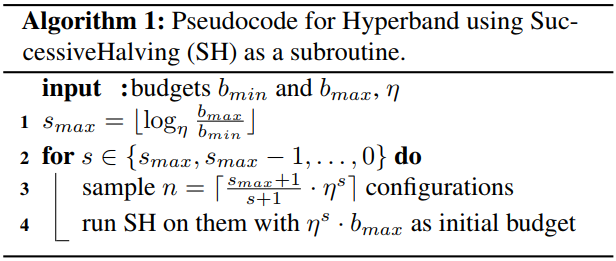The second part BO (Bayesian Optimization) The BO part of BOHB closely resembles TPE with one major difference: It opted for a single multidimensional KDE compared to the hierarchy of one-dimensional KDEs used in TPE in order to better handle interaction effects in the input space. Tree Parzen Estimator(TPE): uses a KDE (kernel density estimator) to model the densities.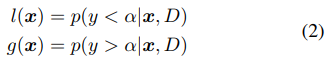To fit useful KDEs, we require a minimum number of data points Nmin; this is set to d + 1 for our experiments, where d is the number of hyperparameters. To build a model as early as possible, we do not wait until Nb = |Db|, where the number of observations for budget b is large enough to satisfy q · Nb ≥ Nmin. Instead, after initializing with Nmin + 2 random configurations, we choose the best and worst configurations, respectively, to model the two densities. Note that it also samples a constant fraction named random fraction of the configurations uniformly at random.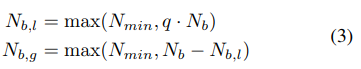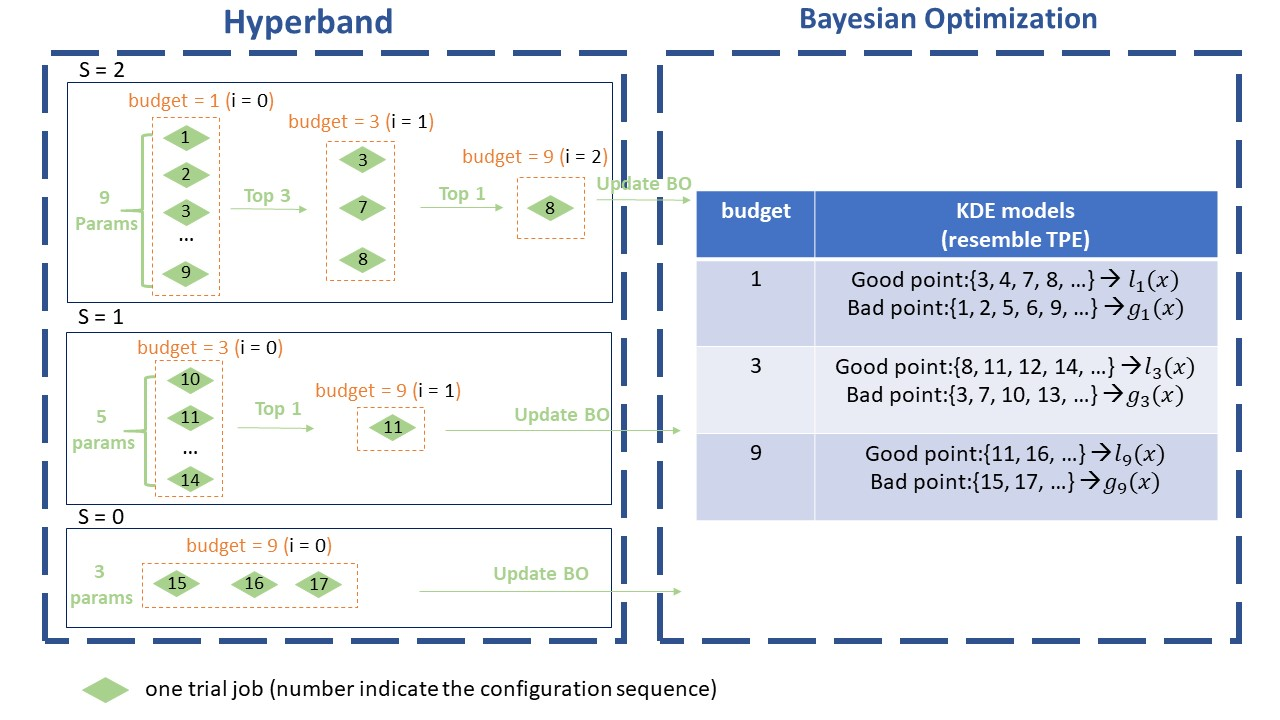The above image shows the workflow of BOHB. Here set max_budget = 9, min_budget = 1, eta = 3, others as default. In this case, s_max = 2, so we will continuously run the {s=2, s=1, s=0, s=2, s=1, s=0, ...} cycle. In each stage of SuccessiveHalving (the orange box), it will pick the top 1/eta configurations and run them again with more budget, repeating the SuccessiveHalving stage until the end of this iteration. At the same time, it collects the configurations, budgets and final metrics of each trial and use these to build a multidimensional KDEmodel with the key "budget". Multidimensional KDE is used to guide the selection of configurations for the next iteration. The sampling procedure (using Multidimensional KDE to guide selection) is summarized by the pseudocode below.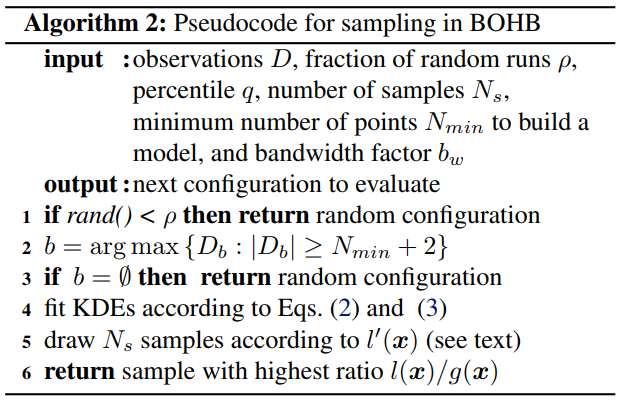Here is a simple experiment which tunes MNIST with BOHB. Code implementation: examples/trials/mnist-advisor The following is the experimental final results: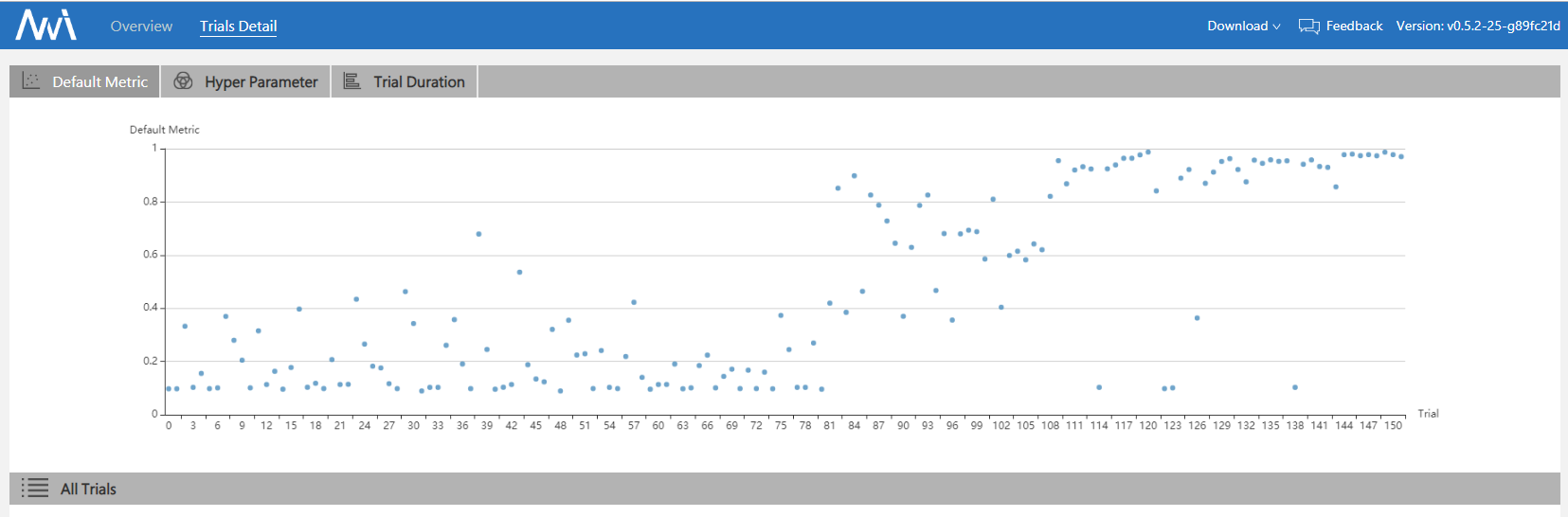More experimental results can be found in the reference paper. It shows that BOHB makes good use of previous results and has a balanced trade-off in exploration and exploitation.

### DNGO Tuner¶

class nni.algorithms.hpo.dngo_tuner.DNGOTuner(optimize_mode='maximize', sample_size=1000, trials_per_update=20, num_epochs_per_training=500)[源代码]

Use neural networks as an alternative to GPs to model distributions over functions in bayesian optimization.

• optimize (maximize | minimize, default = maximize) -- If 'maximize', the tuner will target to maximize metrics. If 'minimize', the tuner will target to minimize metrics.

• sample_size (int, default = 1000) -- Number of samples to select in each iteration. The best one will be picked from the samples as the next trial.

• trials_per_update (int, default = 20) -- Number of trials to collect before updating the model.

• num_epochs_per_training (int, default = 500) -- Number of epochs to train DNGO model.

### Evolution Tuner¶

class nni.algorithms.hpo.evolution_tuner.EvolutionTuner(optimize_mode='maximize', population_size=32)[源代码]

Naive Evolution comes from Large-Scale Evolution of Image Classifiers It randomly initializes a population based on the search space. For each generation, it chooses better ones and does some mutation. (e.g., changes a hyperparameter, adds/removes one layer, etc.) on them to get the next generation. Naive Evolution requires many trials to works but it’s very simple and it’s easily expanded with new features.

config.tuner.name = 'Evolution'
config.tuner.class_args = {
'optimize_mode': 'maximize',
'population_size': 100
}


• optimize_mode (str) -- Optimize mode, 'maximize' or 'minimize'. If 'maximize', the tuner will try to maximize metrics. If 'minimize', the tuner will try to minimize metrics.

• population_size (int) -- The initial size of the population (trial num) in the evolution tuner(default=32). The larger population size, the better evolution performance. It's suggested that population_size be much larger than concurrency so users can get the most out of the algorithm. And at least concurrency, or the tuner will fail on its first generation of parameters.

### GP Tuner¶

class nni.algorithms.hpo.gp_tuner.GPTuner(optimize_mode='maximize', utility='ei', kappa=5, xi=0, nu=2.5, alpha=1e-06, cold_start_num=10, selection_num_warm_up=100000, selection_num_starting_points=250)[源代码]

GP tuner is a Bayesian Optimization method where Gaussian Process is used for modeling loss functions.

Bayesian optimization works by constructing a posterior distribution of functions (a Gaussian Process) that best describes the function you want to optimize. As the number of observations grows, the posterior distribution improves, and the algorithm becomes more certain of which regions in parameter space are worth exploring and which are not.

GP tuner is designed to minimize/maximize the number of steps required to find a combination of parameters that are close to the optimal combination. To do so, this method uses a proxy optimization problem (finding the maximum of the acquisition function) that, albeit still a hard problem, is cheaper (in the computational sense) to solve, and it's amenable to common tools. Therefore, Bayesian Optimization is suggested for situations where sampling the function to be optimized is very expensive.

Note that the only acceptable types in the search space are randint, uniform, quniform, loguniform, qloguniform, and numerical choice.

This optimization approach is described in Section 3 of the paper Algorithms for Hyper-Parameter Optimization ( Bergstra et al. ).

config.tuner.name = 'GP'
config.tuner.class_args = {
'optimize_mode': 'maximize',
'utility': 'ei',
'kappa': 5.0,
'xi': 0.0,
'nu': 2.5,
'alpha': 1e-6,
'cold_start_num': 10,
'selection_num_warm_up': 100000,
'selection_num_starting_points': 250
}


• optimize_mode (str) -- Optimize mode, 'maximize' or 'minimize', by default 'maximize'

• utility (str) -- Utility function (also called 'acquisition funcition') to use, which can be 'ei', 'ucb' or 'poi'. By default 'ei'.

• kappa (float) -- Value used by utility function 'ucb'. The bigger kappa is, the more the tuner will be exploratory. By default 5.

• xi (float) -- Used by utility function 'ei' and 'poi'. The bigger xi is, the more the tuner will be exploratory. By default 0.

• nu (float) -- Used to specify Matern kernel. The smaller nu, the less smooth the approximated function is. By default 2.5.

• alpha (float) -- Used to specify Gaussian Process Regressor. Larger values correspond to increased noise level in the observations. By default 1e-6.

• cold_start_num (int) -- Number of random exploration to perform before Gaussian Process. By default 10.

• selection_num_warm_up (int) -- Number of random points to evaluate for getting the point which maximizes the acquisition function. By default 100000

• selection_num_starting_points (int) -- Number of times to run L-BFGS-B from a random starting point after the warmup. By default 250.

### Grid Search Tuner¶

class nni.algorithms.hpo.gridsearch_tuner.GridSearchTuner(optimize_mode=None)[源代码]

Grid search tuner divides search space into evenly spaced grid, and performs brute-force traverse.

Recommended when the search space is small, or if you want to find strictly optimal hyperparameters.

Implementation

The original grid search approach performs an exhaustive search through a space consists of choice and randint.

NNI's implementation extends grid search to support all search spaces types.

When the search space contains continuous parameters like normal and loguniform, grid search tuner works in following steps:

1. Divide the search space into a grid.

2. Perform an exhaustive searth through the grid.

3. Subdivide the grid into a finer-grained new grid.

4. Goto step 2, until experiment end.

As a deterministic algorithm, grid search has no argument.

config.tuner.name = 'GridSearch'


### Hyperband Tuner¶

Hyperband is a multi-fidelity hyperparameter tuning algorithm based on successive halving.

The basic idea of Hyperband is to create several buckets, each having n randomly generated hyperparameter configurations, each configuration using r resources (e.g., epoch number, batch number). After the n configurations are finished, it chooses the top n/eta configurations and runs them using increased r*eta resources. At last, it chooses the best configuration it has found so far. Please refer to the paper Li et al. for detailed algorithm.

config.tuner.name = 'Hyperband'
config.tuner.class_args = {
'optimize_mode': 'maximize',
'R': 60,
'eta': 3
}


Note that once you use Advisor, you are not allowed to add a Tuner and Assessor spec in the config file. When Hyperband is used, the dict returned by nni.get_next_parameter() one more key called TRIAL_BUDGET besides the hyperparameters and their values. With this TRIAL_BUDGET, users can control in trial code how long a trial runs by following the suggested trial budget from Hyperband. TRIAL_BUDGET is a relative number, users can interpret them as number of epochs, number of mini-batches, running time, etc.

Here is a concrete example of R=81 and eta=3:

s=4

s=3

s=2

s=1

s=0

i

n r

n r

n r

n r

n r

0

81 1

27 3

9 9

6 27

5 81

1

27 3

9 9

3 27

2 81

2

9 9

3 27

1 81

3

3 27

1 81

4

1 81

s means bucket, n means the number of configurations that are generated, the corresponding r means how many budgets these configurations run. i means round, for example, bucket 4 has 5 rounds, bucket 3 has 4 rounds.

• optimize_mode (str) -- Optimize mode, 'maximize' or 'minimize'.

• R (int) -- The maximum amount of budget that can be allocated to a single configuration. Here, trial budget could mean the number of epochs, number of mini-batches, etc., depending on how users interpret it. Each trial should use TRIAL_BUDGET to control how long it runs.

• eta (int) -- The variable that controls the proportion of configurations discarded in each round of SuccessiveHalving. 1/eta configurations will survive and rerun using more budgets in each round.

• exec_mode (str) -- Execution mode, 'serial' or 'parallelism'. If 'parallelism', the tuner will try to use available resources to start new bucket immediately. If 'serial', the tuner will only start new bucket after the current bucket is done.

First, Hyperband an example of how to write an autoML algorithm based on MsgDispatcherBase, rather than based on Tuner and Assessor. Hyperband is implemented in this way because it integrates the functions of both Tuner and Assessor,thus, we call it Advisor.

Second, this implementation fully leverages Hyperband's internal parallelism. Specifically, the next bucket is not started strictly after the current bucket. Instead, it starts when there are available resources. If you want to use full parallelism mode, set exec_mode to parallelism.

Or if you want to set exec_mode with serial according to the original algorithm. In this mode, the next bucket will start strictly after the current bucket.

parallelism mode may lead to multiple unfinished buckets, in contrast, there is at most one unfinished bucket under serial mode. The advantage of parallelism mode is to make full use of resources, which may reduce the experiment duration multiple times.

### Metis Tuner¶

class nni.algorithms.hpo.metis_tuner.MetisTuner(optimize_mode='maximize', no_resampling=True, no_candidates=False, selection_num_starting_points=600, cold_start_num=10, exploration_probability=0.9)[源代码]

Metis tuner offers several benefits over other tuning algorithms. While most tools only predict the optimal configuration, Metis gives you two outputs, a prediction for the optimal configuration and a suggestion for the next trial. No more guess work!

While most tools assume training datasets do not have noisy data, Metis actually tells you if you need to resample a particular hyper-parameter.

While most tools have problems of being exploitation-heavy, Metis' search strategy balances exploration, exploitation, and (optional) resampling.

Metis belongs to the class of sequential model-based optimization (SMBO) algorithms and it is based on the Bayesian Optimization framework. To model the parameter-vs-performance space, Metis uses both a Gaussian Process and GMM. Since each trial can impose a high time cost, Metis heavily trades inference computations with naive trials. At each iteration, Metis does two tasks (refer to Li et al. for details):

1. It finds the global optimal point in the Gaussian Process space. This point represents the optimal configuration.

2. It identifies the next hyper-parameter candidate. This is achieved by inferring the potential information gain of exploration, exploitation, and resampling.

Note that the only acceptable types in the search space are quniform, uniform, randint, and numerical choice.

config.tuner.name = 'Metis'
config.tuner.class_args = {
'optimize_mode': 'maximize'
}


• optimize_mode (str) -- optimize_mode is a string that including two mode "maximize" and "minimize"

• no_resampling (bool) -- True or False. Should Metis consider re-sampling as part of the search strategy? If you are confident that the training dataset is noise-free, then you do not need re-sampling.

• no_candidates (bool) -- True or False. Should Metis suggest parameters for the next benchmark? If you do not plan to do more benchmarks, Metis can skip this step.

• selection_num_starting_points (int) -- How many times Metis should try to find the global optimal in the search space? The higher the number, the longer it takes to output the solution.

• cold_start_num (int) -- Metis need some trial result to get cold start. when the number of trial result is less than cold_start_num, Metis will randomly sample hyper-parameter for trial.

• exploration_probability (float) -- The probability of Metis to select parameter from exploration instead of exploitation.

### PBT Tuner¶

class nni.algorithms.hpo.pbt_tuner.PBTTuner(optimize_mode='maximize', all_checkpoint_dir=None, population_size=10, factor=0.2, resample_probability=0.25, fraction=0.2)[源代码]

Population Based Training (PBT) comes from Population Based Training of Neural Networks. It's a simple asynchronous optimization algorithm which effectively utilizes a fixed computational budget to jointly optimize a population of models and their hyperparameters to maximize performance. Importantly, PBT discovers a schedule of hyperparameter settings rather than following the generally sub-optimal strategy of trying to find a single fixed set to use for the whole course of training.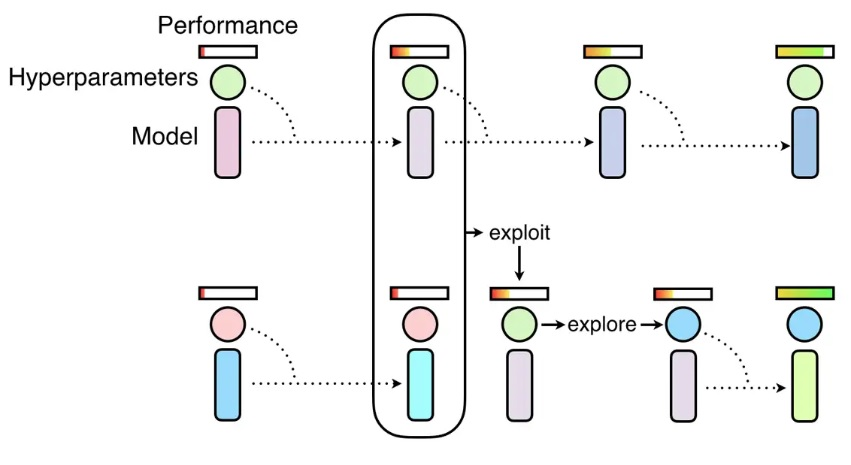PBT tuner initializes a population with several trials (i.e., population_size). There are four steps in the above figure, each trial only runs by one step. How long is one step is controlled by trial code, e.g., one epoch. When a trial starts, it loads a checkpoint specified by PBT tuner and continues to run one step, then saves checkpoint to a directory specified by PBT tuner and exits. The trials in a population run steps synchronously, that is, after all the trials finish the i-th step, the (i+1)-th step can be started. Exploitation and exploration of PBT are executed between two consecutive steps.

Two important steps to follow if you are trying to use PBT tuner:

1. Provide checkpoint directory. Since some trials need to load other trial's checkpoint, users should provide a directory (i.e., all_checkpoint_dir) which is accessible by every trial. It is easy for local mode, users could directly use the default directory or specify any directory on the local machine. For other training services, users should follow the document of those training services to provide a directory in a shared storage, such as NFS, Azure storage.

2. Modify your trial code. Before running a step, a trial needs to load a checkpoint, the checkpoint directory is specified in hyper-parameter configuration generated by PBT tuner, i.e., params['load_checkpoint_dir']. Similarly, the directory for saving checkpoint is also included in the configuration, i.e., params['save_checkpoint_dir']. Here, all_checkpoint_dir is base folder of load_checkpoint_dir and save_checkpoint_dir whose format is all_checkpoint_dir/<population-id>/<step>.

params = nni.get_next_parameter()
# the path of the checkpoint to load
# load checkpoint from load_path
...
# run one step
...
# the path for saving a checkpoint
save_path = os.path.join(params['save_checkpoint_dir'], 'model.pth')
# save checkpoint to save_path
...


The complete example code can be found here.

• optimize_mode (maximize or minimize, default: maximize) -- If maximize, the tuner will target to maximize metrics. If minimize, the tuner will target to minimize metrics.

• all_checkpoint_dir (str) -- Directory for trials to load and save checkpoint. If not specified, the directory would be ~/nni/checkpoint/. Note that if the experiment is not local mode, users should provide a path in a shared storage which can be accessed by all the trials.

• population_size (int, default = 10) -- Number of trials in a population. Each step has this number of trials. In our implementation, one step is running each trial by specific training epochs set by users.

• factor (float, default = (1.2, 0.8)) -- Factors for perturbation of hyperparameters.

• resample_probability (float, default = 0.25) -- Probability for resampling.

• fraction (float, default = 0.2) -- Fraction for selecting bottom and top trials.

Below is an example of PBT tuner configuration in experiment config file.

tuner:
name: PBT
classArgs:
optimize_mode: maximize
all_checkpoint_dir: /the/path/to/store/checkpoints
population_size: 10


Assessor is not allowed if PBT tuner is used.

### PPO Tuner¶

class nni.algorithms.hpo.ppo_tuner.PPOTuner(optimize_mode, trials_per_update=20, epochs_per_update=4, minibatch_size=4, ent_coef=0.0, lr=0.0003, vf_coef=0.5, max_grad_norm=0.5, gamma=0.99, lam=0.95, cliprange=0.2)[源代码]

PPOTuner, the implementation inherits the main logic of the implementation ppo2 from openai and is adapted for NAS scenario. It uses lstm for its policy network and value network, policy and value share the same network.

• optimize_mode (str) -- maximize or minimize

• trials_per_update (int) -- Number of trials to have for each model update

• epochs_per_update (int) -- Number of epochs to run for each model update

• minibatch_size (int) -- Minibatch size (number of trials) for the update

• ent_coef (float) -- Policy entropy coefficient in the optimization objective

• lr (float) -- Learning rate of the model (lstm network), constant

• vf_coef (float) -- Value function loss coefficient in the optimization objective

• gamma (float) -- Discounting factor

• lam (float) -- Advantage estimation discounting factor (lambda in the paper)

• cliprange (float) -- Cliprange in the PPO algorithm, constant

### Random Tuner¶

class nni.algorithms.hpo.random_tuner.RandomTuner(seed=None, optimize_mode=None)[源代码]

A naive tuner that generates fully random hyperparameters.

config.tuner.name = 'Random'
config.tuner.class_args = {
'seed': 100
}


seed (int | None) -- The random seed.

### SMAC Tuner¶

class nni.algorithms.hpo.smac_tuner.SMACTuner(optimize_mode='maximize', config_dedup=False)[源代码]

SMAC is based on Sequential Model-Based Optimization (SMBO). It adapts the most prominent previously used model class (Gaussian stochastic process models) and introduces the model class of random forests to SMBO in order to handle categorical parameters.

The SMAC supported by nni is a wrapper on the SMAC3 github repo, following NNI tuner interface nni.tuner.Tuner. For algorithm details of SMAC, please refer to the paper Hutter et al..

Note that SMAC on nni only supports a subset of the types in search space: choice, randint, uniform, loguniform, and quniform.

Note that SMAC needs additional installation using the following command:

pip install nni[SMAC]


swig is required for SMAC. for Ubuntu swig can be installed with apt.

config.tuner.name = 'SMAC'
config.tuner.class_args = {
'optimize_mode': 'maximize'
}


• optimize_mode (str) -- Optimize mode, 'maximize' or 'minimize', by default 'maximize'

• config_dedup (bool) -- If True, the tuner will not generate a configuration that has been already generated. If False, a configuration may be generated twice, but it is rare for relatively large search space.

### TPE Tuner¶

class nni.algorithms.hpo.tpe_tuner.TpeTuner(optimize_mode='minimize', seed=None, tpe_args=None)[源代码]

Tree-structured Parzen Estimator (TPE) tuner.

TPE is a lightweight tuner that has no extra dependency and supports all search space types, designed to be the default tuner.

It has the drawback that TPE cannot discover relationship between different hyperparameters.

Implementation

TPE is an SMBO algorithm. It models P(x|y) and P(y) where x represents hyperparameters and y the evaluation result. P(x|y) is modeled by transforming the generative process of hyperparameters, replacing the distributions of the configuration prior with non-parametric densities.

## minimal config ##

config.tuner.name = 'TPE'
config.tuner.class_args = {
'optimize_mode': 'maximize'
}

## advanced config ##

config.tuner.name = 'TPE'
config.tuner.class_args = {
'optimize_mode': maximize,
'seed': 12345,
'tpe_args': {
'constant_liar_type': 'mean',
'n_startup_jobs': 10,
'n_ei_candidates': 20,
'linear_forgetting': 100,
'prior_weight': 0,
'gamma': 0.5
}
}


• optimze_mode (Literal['minimize', 'maximize']) -- Whether optimize to minimize or maximize trial result.

• seed (int | None) -- The random seed.

• tpe_args (dict[str, Any] | None) -- Advanced users can use this to customize TPE tuner. See TpeArguments for details.

class nni.algorithms.hpo.tpe_tuner.TpeArguments(constant_liar_type='best', n_startup_jobs=20, n_ei_candidates=24, linear_forgetting=25, prior_weight=1.0, gamma=0.25)[源代码]

Hyperparameters of TPE algorithm itself.

To avoid confusing with trials' hyperparameters to be tuned, these are called "arguments" here.

• constant_liar_type (Literal['best', 'worst', 'mean'] | None) --

TPE algorithm itself does not support parallel tuning. This parameter specifies how to optimize for trial_concurrency > 1.

None (or "null" in YAML) means do not optimize. This is the default behavior in legacy version.

How each liar works is explained in paper's section 6.1. In general "best" suit for small trial number and "worst" suit for large trial number. (experiment result)

• n_startup_jobs (int) -- The first N hyperparameters are generated fully randomly for warming up. If the search space is large, you can increase this value. Or if max_trial_number is small, you may want to decrease it.

• n_ei_candidates (int) -- For each iteration TPE samples EI for N sets of parameters and choose the best one. (loosely speaking)

• linear_forgetting (int) -- TPE will lower the weights of old trials. This controls how many iterations it takes for a trial to start decay.

• prior_weight (float) --

TPE treats user provided search space as prior. When generating new trials, it also incorporates the prior in trial history by transforming the search space to one trial configuration (i.e., each parameter of this configuration chooses the mean of its candidate range). Here, prior_weight determines the weight of this trial configuration in the history trial configurations.

With prior weight 1.0, the search space is treated as one good trial. For example, "normal(0, 1)" effectly equals to a trial with x = 0 which has yielded good result.

• gamma (float) -- Controls how many trials are considered "good". The number is calculated as "min(gamma * sqrt(N), linear_forgetting)".

## Assessors¶

### Curve Fitting Assessor¶

class nni.algorithms.hpo.curvefitting_assessor.CurvefittingAssessor(epoch_num=20, start_step=6, threshold=0.95, gap=1)[源代码]

CurvefittingAssessor uses learning curve fitting algorithm to predict the learning curve performance in the future.

The intermediate result must be accuracy. Curve fitting does not support minimizing loss.

Curve fitting assessor is an LPA (learning, predicting, assessing) algorithm. It stops a pending trial X at step S if the trial's forecast result at target step is convergence and lower than the best performance in the history.

config.assessor.name = 'Curvefitting'
config.assessor.class_args = {
'epoch_num': 20,
'start_step': 6,
'threshold': 9,
'gap': 1,
}


• epoch_num (int) --

The total number of epochs.

We need to know the number of epochs to determine which points we need to predict.

• start_step (int) -- A trial is determined to be stopped or not only after receiving start_step number of intermediate results.

• threshold (float) --

The threshold that we use to decide to early stop the worst performance curve.

For example: if threshold = 0.95, and the best performance in the history is 0.9, then we will stop the trial who's predicted value is lower than 0.95 * 0.9 = 0.855.

• gap (int) --

The gap interval between assessor judgements.

For example: if gap = 2, start_step = 6, then we will assess the result when we get 6, 8, 10, 12, ... intermediate results.

### Median Stop Assessor¶

class nni.algorithms.hpo.medianstop_assessor.MedianstopAssessor(optimize_mode='maximize', start_step=0)[源代码]

The median stopping rule stops a pending trial X at step S if the trial’s best objective value by step S is strictly worse than the median value of the running averages of all completed trials’ objectives reported up to step S

config.assessor.name = 'Medianstop'
config.assessor.class_args = {
'optimize_mode': 'maximize',
'start_step': 5
}


• optimize_mode (Literal['minimize', 'maximize']) -- Whether optimize to minimize or maximize trial result.

• start_step (int) -- A trial is determined to be stopped or not only after receiving start_step number of reported intermediate results.

## Customization¶

class nni.assessor.AssessResult(value)[源代码]

Enum class for Assessor.assess_trial() return value.

The trial works poorly and should be early stopped.

Good = True

The trial works well.

class nni.assessor.Assessor[源代码]

Assessor analyzes trial's intermediate results (e.g., periodically evaluated accuracy on test dataset) to tell whether this trial can be early stopped or not.

This is the abstract base class for all assessors. Early stopping algorithms should inherit this class and override assess_trial() method, which receives intermediate results from trials and give an assessing result.

If assess_trial() returns AssessResult.Bad for a trial, it hints NNI framework that the trial is likely to result in a poor final accuracy, and therefore should be killed to save resource.

If an assessor want's to be notified when a trial ends, it can also override trial_end().

To write a new assessor, you can reference MedianstopAssessor's code as an example.

assess_trial(trial_job_id, trial_history)[源代码]

Abstract method for determining whether a trial should be killed. Must override.

The NNI framework has little guarantee on trial_history. This method is not guaranteed to be invoked for each time trial_history get updated. It is also possible that a trial's history keeps updating after receiving a bad result. And if the trial failed and retried, trial_history may be inconsistent with its previous value.

The only guarantee is that trial_history is always growing. It will not be empty and will always be longer than previous value.

This is an example of how assess_trial() get invoked sequentially:

trial_job_id | trial_history   | return value
------------ | --------------- | ------------
Trial_A      | [1.0, 2.0]      | Good
Trial_B      | [1.5, 1.3]      | Bad
Trial_B      | [1.5, 1.3, 1.9] | Good
Trial_A      | [0.9, 1.8, 2.3] | Good


• trial_job_id (str) -- Unique identifier of the trial.

• trial_history (list) -- Intermediate results of this trial. The element type is decided by trial code.

AssessResult

Internal API under revising, not recommended for end users.

save_checkpoint()[源代码]

Internal API under revising, not recommended for end users.

trial_end(trial_job_id, success)[源代码]

Abstract method invoked when a trial is completed or terminated. Do nothing by default.

• trial_job_id (str) -- Unique identifier of the trial.

• success (bool) -- True if the trial successfully completed; False if failed or terminated.

class nni.tuner.Tuner[源代码]

Tuner is an AutoML algorithm, which generates a new configuration for the next try. A new trial will run with this configuration.

This is the abstract base class for all tuners. Tuning algorithms should inherit this class and override update_search_space(), receive_trial_result(), as well as generate_parameters() or generate_multiple_parameters().

After initializing, NNI will first call update_search_space() to tell tuner the feasible region, and then call generate_parameters() one or more times to request for hyper-parameter configurations.

The framework will train several models with given configuration. When one of them is finished, the final accuracy will be reported to receive_trial_result(). And then another configuration will be reqeusted and trained, util the whole experiment finish.

If a tuner want's to know when a trial ends, it can also override trial_end().

Tuners use parameter ID to track trials. In tuner context, there is a one-to-one mapping between parameter ID and trial. When the framework ask tuner to generate hyper-parameters for a new trial, an ID has already been assigned and can be recorded in generate_parameters(). Later when the trial ends, the ID will be reported to trial_end(), and receive_trial_result() if it has a final result. Parameter IDs are unique integers.

The type/format of search space and hyper-parameters are not limited, as long as they are JSON-serializable and in sync with trial code. For HPO tuners, however, there is a widely shared common interface, which supports choice, randint, uniform, and so on. See docs/en_US/Tutorial/SearchSpaceSpec.md for details of this interface.

[WIP] For advanced tuners which take advantage of trials' intermediate results, an Advisor interface is under development.

Builtin, HyperoptTuner, EvolutionTuner, SMACTuner, GridSearchTuner, NetworkMorphismTuner, MetisTuner, PPOTuner, GPTuner

generate_multiple_parameters(parameter_id_list, **kwargs)[源代码]

Callback method which provides multiple sets of hyper-parameters.

This method will get called when the framework is about to launch one or more new trials.

If user does not override this method, it will invoke generate_parameters() on each parameter ID.

See generate_parameters() for details.

User code must override either this method or generate_parameters().

• parameter_id_list (list of int) -- Unique identifiers for each set of requested hyper-parameters. These will later be used in receive_trial_result().

• **kwargs -- Unstable parameters which should be ignored by normal users.

List of hyper-parameters. An empty list indicates there are no more trials.

list

generate_parameters(parameter_id, **kwargs)[源代码]

Abstract method which provides a set of hyper-parameters.

This method will get called when the framework is about to launch a new trial, if user does not override generate_multiple_parameters().

The return value of this method will be received by trials via nni.get_next_parameter(). It should fit in the search space, though the framework will not verify this.

User code must override either this method or generate_multiple_parameters().

• parameter_id (int) -- Unique identifier for requested hyper-parameters. This will later be used in receive_trial_result().

• **kwargs -- Unstable parameters which should be ignored by normal users.

The hyper-parameters, a dict in most cases, but could be any JSON-serializable type when needed.

any

nni.NoMoreTrialError -- If the search space is fully explored, tuner can raise this exception.

import_data(data)[源代码]

Internal API under revising, not recommended for end users.

Internal API under revising, not recommended for end users.

Abstract method invoked when a trial reports its final result. Must override.

This method only listens to results of algorithm-generated hyper-parameters. Currently customized trials added from web UI will not report result to this method.

save_checkpoint()[源代码]

Internal API under revising, not recommended for end users.

trial_end(parameter_id, success, **kwargs)[源代码]

Abstract method invoked when a trial is completed or terminated. Do nothing by default.

• parameter_id (int) -- Unique identifier for hyper-parameters used by this trial.

• success (bool) -- True if the trial successfully completed; False if failed or terminated.

• **kwargs -- Unstable parameters which should be ignored by normal users.

update_search_space(search_space)[源代码]

Abstract method for updating the search space. Must override.

Tuners are advised to support updating search space at run-time. If a tuner can only set search space once before generating first hyper-parameters, it should explicitly document this behaviour.

search_space (Dict[str, _ParameterSearchSpace]) -- JSON object defined by experiment owner.